Next: ELASTIC WAVES Up: Mathematical physics in stratified Previous: SOURCE-RECEIVER RECIPROCITY

# CONSERVATION PRINCIPLES AND MODE ORTHOGONALITY

We showed earlier how problems in stratified media reduce to a first-order matrix differential equation of the form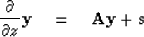(1)
It turns out that many problems in the form of (9-5-1) can be reformulated into what we will call the Atkinson form. It is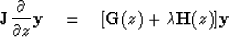(2)
whereis a skew-Hermitian matrix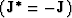independent of z,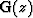and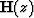are Hermitian matrices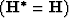,andis a scalar which will come to play the role of an eigenvalue. For example, in acoustics we have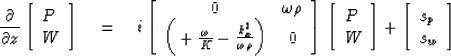(3)
which can be premultiplied by a skew-Hermitian matrix to give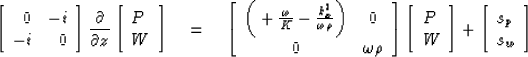(4)
The significant thing about (9-5-4) is that the operators are self-adjoint, meaning that the right-hand matrix is Hermitian and so is the left-hand operator. To understand why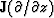is Hermitian, write it out as a difference approximation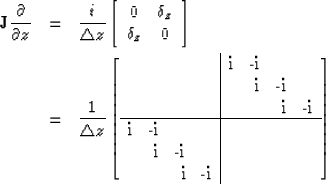(5)
Inspecting (9-5-5) we see that it is two rows short of being square. Choosing two boundary conditions will be like obtaining two more rows. Clearly (9-5-5) is so close to being Hermitian that two more rows can be chosen to make it Hermitian. For example, the two rows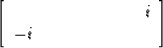could be squeezed between the top and bottom halves of (9-5-5). Since the operator (9-5-5) can be made Hermitian by choice of suitable boundary conditions and since the other operators in (9-5-4) are already Hermitian, it seems that the Atkinson form applies to physical problems in which the reciprocity principle is applicable. Reciprocity does apply to most geophysical prospecting problems. A simple physical situation in which reciprocity does not apply is sound waves in a windy atmosphere. Physically it is because waves go more slowly upwind than downwind, and mathematically it is because nomatrix can be found to convert (9-5-1) into the form (9-5-2). Only in a source-free region can we convert (9-5-1) to (9-5-2). If we choose to let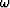play the role of the eigenvalue, then taking source terms to be zero we split (9-5-4) into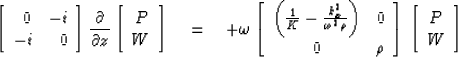(6)
Herehas turned out to vanish and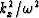,which is proportional to the sine of the incident angle, is to be regarded as a constant for variable values of the eigenvalue.Alternatively, we could choose -k2x to be the eigenvalue, and then (9-5-4) would become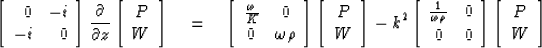(7)
Obviously, still another possibility is to let the angle variable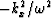be the eigenvalue for fixed.

The Atkinson form (9-5-2) leads directly to various conservation principles. Let us computer the vertical derivative of the quadratic form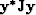.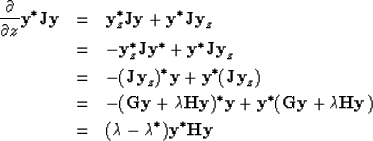(8)
Very often we take the eigenvalues,-k2x, orto be real, and in such a case we have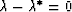and (9-5-8) shows theis a quadratic function of the wave variables which is invariant with z. In the acoustic example, this quadratic invariant is proportional to the energy flux. Specifically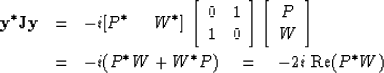(9)
If we wish to consider a complex frequency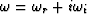, then in the first acoustic example (9-5-6) equation (9-5-8) becomes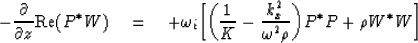(10)
Noting that if P and W have time dependence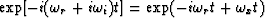, then quadratics like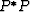and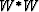have time dependence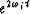and we see that the multiplier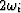can be regarded as a time derivative. Hence (9-5-10) becomes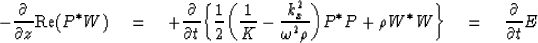(11)
Equation (9-5-11) is interpreted as saying that the time derivative of the energy density E at a point is proportional to the negative of the divergence of energy flux at that point. In other problems the quadratic forms need not always turn out to involve energy. Sometimes momentum in involved.

A well-known theorem in matrix theory is that Hermitian matrices have real eigenvalues. Why then did we consider the possibility of a complex eigenvalue in (9-5-8)? The answer is that the finite difference operator matrix need not be chosen to have boundary conditions which make the operators Hermitian. In particular, for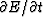to be nonzero, energy must leak in or out at a boundary.

Now, let us suppose boundary conditions have been chosen to makesymmetric so the eigenvalues become real. Let yn(z) be a solution to (9-5-2) with eigenvalue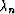,and let ym(z) be another solution with a different eigenvalue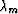.The reasoning which led up to (9-5-8) can be used to obtain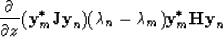(12)
Integrating through z from za to zb, we have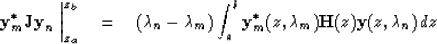(13)
If boundary conditions have been chosen so that no energy gets in or out at za and zb, then the left-hand side vanishes. Since by hypothesis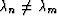we must have the right-hand integral vanishing. This states the orthogonality of the two solutions (called the two modes) and the idea is the same as the orthogonality of eigenvectors of the Hermitian difference operator matrices. The orthogonality of these functions is frequently useful in theoretical and computational work.

## EXERCISES:

1. Show that application of (9-5-8) to (9-5-7) leads to a definition of horizontal energy flux. You may wish to take kx = kr + iki and assume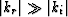.Next: ELASTIC WAVES Up: Mathematical physics in stratified Previous: SOURCE-RECEIVER RECIPROCITY
Stanford Exploration Project
10/30/1997